# Test: RCC- 2

## 20 Questions MCQ Test Mock Test Series of SSC JE Civil Engineering | Test: RCC- 2

Description
Attempt Test: RCC- 2 | 20 questions in 12 minutes | Mock test for SSC preparation | Free important questions MCQ to study Mock Test Series of SSC JE Civil Engineering for SSC Exam | Download free PDF with solutions
QUESTION: 1

### If the permissible compressive and tensile stresses in a single reinforced beam are 50 kg/cm2 and 1400 kg/cm2 respectively and the modular ratio is 18, the percentage of the steel required for an economic section, is

Solution:

Taking Moment of area of tension and compression zone for a singly reinforced beam: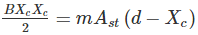Where,

Xc = critical depth or economic depth of neutral axis

m = modular ratio

Ast = area of steel required for balanced section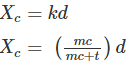m = 18

c = 50 kg/cm2

t = 1400 kg/cm2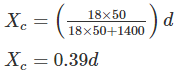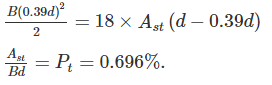QUESTION: 2

### Though the effective depth of a T-beam is the distance between the top compression edge to the centre of the tensile reinforcement, for heavy loads it is taken as:

Solution:

For all cases, Effective depth of the beam cross-section is to be taken as: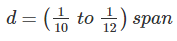QUESTION: 3

### If the diameter of main reinforcement in a slab is 16 mm, the concrete cover to main bar according to IS 456:2000 is:

Solution:

According to IS 456: 2000, minimum nominal cover to all bars in the slab is 20mm. According to new code, nominal cover does not depend upon the diameter of bar used.

QUESTION: 4

__________ is the type of concrete in which internal stresses are intentionally induced in a planned manner such that the stresses resulting from the superimposed loads get counteracted to a desired degree.

Solution:

The principle behind prestressed concrete is that compressive stresses induced by high-strength steel tendons in a concrete member before loads are applied will balance the tensile stresses imposed in the member during service.

A prestressed concrete member is one in which there have been introduced internal stresses of such a magnitude, and also distribution, that the stresses resulting from external loading are counteracted to a desired degree. By doing this, Nearly the full capacity of the concrete in compression can be used over entire depth under full loading.

QUESTION: 5

Span to effective depth ratio for a continuous beam with steel grade of Fe 415 should not be more than: -

Solution:

It is necessary to impose a check on the magnitude of deflection in a structural member:

a) To ensure that the extent of deflection does not adversely affect the appearance or efficiency of the structure or finishes or partition etc.

b) To prevent structural behaviour of the member being different from the assumption made in the design. As per IS 456:2000, for beams and slabs, the vertical deflection limits may be assumed to be satisfied, provided that the span to depth ratio are not greater than the values obtained as below: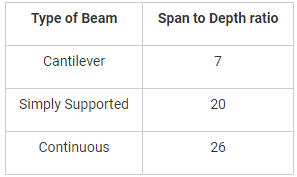QUESTION: 6

The working stress method of design of RC members is known as:-

Solution:

Working stress method: working stress method is based on elastic theory. Concrete and steel are assumed to act together elastically and follow hook’s law. This method follows a deterministic approach as it assumes that the loads, factor of safety and permissible stresses are accurately known. In this method material strengths are not fully utilised in designing the member.

Limit state method: This method follows a non-deterministic approach as it adopts probable loads and probable strengths of materials as per actual or based on experience or observations depending upon the situations. Material strengths are fully utilised in designing the member.

QUESTION: 7

The time in minutes, for the member to reach the limit state of structural inadequacy in a standard fire test is known as:

Solution:

As per Bureau of Indian standards,

Period of structural adequacy under fire: The time (t), in minutes, for the member to reach the limit state of structural inadequacy in a standard fire test.

QUESTION: 8

Choose the correct statement regarding reinforcement detailing:

Solution:

As per IS 456:2000,

1. Lap splicing of reinforcement bar more than 36 mm in diameter should be avoided. In case such bars have to be lapped then they should be welded. (Reference IS456 clause 25.2.5).

2. Where there are two or more rows of bars, the bars shall be vertically in line and the minimum vertical distance between the bars shall be 15 mm, two third the maximum nominal size of aggregate or maximum size of bars, whichever is greater.

3. The minimum nominal cover for footing shall not be less than 50 mm.

QUESTION: 9

Solution:
QUESTION: 10

The clear distance from the free end of the cantilever to the lateral restraint shall not exceed

Solution:

As per IS 456:2000, slenderness limit for lateral stability of beam shall be: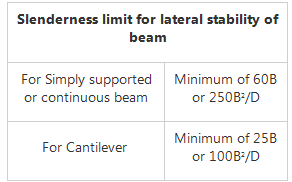QUESTION: 11

For a square column, b × b in size, effective depth of the footing being ‘d’, the punching shear is calculated for an area

Solution:

The critical section for shear is act at a distance of d/2 from the face of the column. So, the total resisting area which resist that punching shear will be

= 4(bd + d2).

QUESTION: 12

The Design ultimate load on the short axially loaded column is computed by which of the following equation?

where,

fck = Characterstics strength of concrete

fy = characterstic strength of steel

Ac = Area of concrete in column cross-section

Asc = Area of steel in column in compression

Solution:

As per IS 456 : 2000 In the Design of compression members the ultimate load on the short axially loaded column is:

Pu = 0.4fck Ac + 0.67fy Asc

where,

fck = Characterstics strength of concrete

fy = characterstic strength of steel

Ac = Area of concrete in column cross-section

Asc = Area of steel in column in compression

QUESTION: 13

Pick up the correct statement from the following.

Solution:

Dead load is the self-weight of the structure and the load which is permanently attached to the structure and will remain throughout the lifetime of the structure.

So at the beginning of design it is known to the designer.

QUESTION: 14

For a continuous slab of 3 m × 3.5 m size, the minimum overall depth of slab to satisfy vertical deflection limits for the grade of steel FE415 is-

Solution:

Explanation :  For high strength deformed bars of grade Fe415:

For mild steel reinforcement.

Continuous slab 32 ≥ L/d

Simply supported slab ≥ L/d

Continuous slab 40 ≥ L/d

where, L is the shorter span of slab

d is overall depth of the beam

32 ≥ 3000/d

d ≥ 9.37 cm = 10 cm

QUESTION: 15

In a rear counterfort retaining wall, the main reinforcement is provided on the

Solution:

Counterforts are firmly attached to the face slab as well as the base slab. The earth pressure acting on the face slab is transferred to the counterfort which deflects as vertical cantilever. The back of rear counterfort comes under tension and the front face is under compression. So the inclined (back) face of the rear counterfort should be provided with main reinforcement.

QUESTION: 16

In a RCC beam of breadth b and overall depth D exceeding 750 mm, side face reinforcement required and the allowable area of maximum tension reinforcement shall be respectively

Solution:

As per IS 456:2000:

1. Side face reinforcement in a RCC beam of breadth b and overall depth D exceeding 750 mm should be 0.1 %.

2. Maximum area of tension reinforcement should not exceed 4%.

QUESTION: 17

Deep beams are designed for

Solution:

Explanation : A beam, whether deep or conventional, is always designed for bending moment, apart from checks for safety in shear, deflections and cracking.

A beam is said to be deep when the ratio of its effective span to overall depth is less than 2 for simply supported beams, or 2.5 for continuous beams. Often the side faces in deep beams may be subjected to torsion under accidental loads or eccentricities. Hence, when the depth of a beam exceeds 750mm, IS 456:2000 recommends providing side face reinforcement along the two faces: total area of this reinforcement should not be less than 0.1℅ of the web area, and should be distributed on the two faces equally with a spacing not exceeding 300mm or web thickness, whichever is lesser.

QUESTION: 18

In R.C footing on soil, the thickness at the edge should not be less than

Solution:

According to the Code (CI. 34.1.2):

Code restricts the minimum thickness at the edge of the footing to 150 mm for footings in general (and to 300 mm in the case of pile caps).

QUESTION: 19

A doubly reinforced concrete beam has effective cover d’ to the centre of compression reinforcement. ‘xu’ is the depth of neutral axis, and d is the effective depth to the centre of tension reinforcement. What is the maximum strain in concrete at the level of compression reinforcement?

Solution:
QUESTION: 20

A reinforced concrete slab is 128 mm thick. The maximum size of reinforcement bar that can be used is:

Solution:

IS 456:2000 limits the maximum diameter of reinforcing bars to 1/8th of the total thickness of the slab.

Dmax = 128/8 = 16 mm; Hence use 16 mm diameter bars.Use Code STAYHOME200 and get INR 200 additional OFF Use Coupon Code